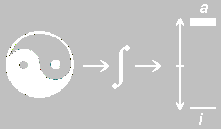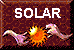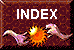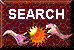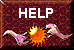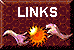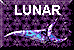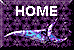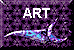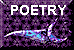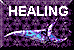# Mensionization in the Emerald Tablet: The Mathematics of Reality

Mensionization In the Emerald Tablet by Charles Higgins

The mathematics used in the manipulation of binary lines is called Mensionization. It is a combination of symbol notation and standard mathematics. Its name is derived from the additive inverse relationship of the dimension. The di portion indicates a two-fold relationship and mension is a derivative of metri, to measure. The two-fold relationship is an oppositional binary.

The complementation of n-binary lines forms one of many functional sequences in Mensionization. Theoretically, there is an infinite number of mensional sequences as can be seen from the diagram of the Mensional Square.

MENSIONAL SQUARE

 0-monomension 0-dimension 0-trimension . . . 0-(n)mension 1-monomension 1-dimension 1-trimension . . . 1-(n)mension 2-monomension 2-dimension 2-trimension . . . 2-(n)mension 3-monomension 3-dimension 3-trimension . . . 3-(n)mension 4-monomension 4-dimension 4-trimension . . . 4-(n)mension * * * . . . * * * * . . . * * * * . . . * r-monomension r-dimension r-trimension . . . r-(n)mension

There is a prominent double sequence in the Mensional Square. Each horizontal row is a sequence and each vertical column is a sequence. The specific sequence we have been discussing is the vertical dimensional sequence because on the surface our universe is a dimensional universe.

In the dimensional sequence, three equations have been previously defined, the 1-dimensional binary, the 2-dimensional complementation and the 3-dimensional quantum complementation.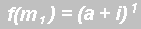1-dimension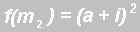2-dimension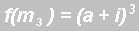3-dimension

The mathematical descriptions do not stop at three dimensions, they include the 4th, 5th, 6th… on to the nth-dimension. Increasing the exponent of the previous equation by one will give the general equation for the next higher dimension.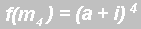4-dimension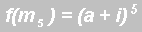5-dimension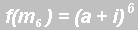6-dimension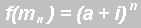n-dimension

This sequence of equations corresponds to the dimensional sequence shown in the Mensional Square. The beginning term of this sequence is not the 1-dimensional equation but the 0-dimensional equation.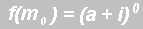0-dimension

The zero exponent equation is the mensional state. It is also a singularity. This state was viewed by the alchemists as a vestige state. It was not material in its nature but was the vestige entity that was the fuel for creation.

The 0-dimension entity is the prime substance referred to in Hermes’ Tablet of Emerald and referenced by the Great Extreme of the I Ching. This prime substance is called a Mension. It is the substance of the “primordial soup” that is in the void or abyss. It is the ONE spoken of in the texts. In the third part of the Musaeum Hermeticum of 1625, which is an explanation of the Tablet of Emerald, are these lines: (Brackets are mine)

The sage [Hermes] tells us that all things were created, and are still generated, from one substance and consist of the same elementary material; and in this first substance God has appointed the four elements, which represent a common material into which to resolve all things.

Also from Hortulanus commentary on the Emerald Tablet:

And as all things have proceeded from one, by the meditation of one. Heere giveth here an example, saying: as all things came from one, to wit, a confused Globe, or masse, by meditation, that is the cogitation and creation of one, that is the omnipotent God: So all thing have sprung, that is, come out from this one thing that is, one confused lump, by Adaptation, that is by the sole commandment of God, and miracle. So our Stone is borne, and come out of one confused mass, containing in it the four Elements, which is created of God, and by his sole miracle our stone is borne.

These excerpts are only two in hundreds of alchemical documents that propose everything that exists is composed of ONE basic primal substance. When the Universal Cause contracted itself and formed the universe, this primal substance was drawn from the void or infinite source and transformed into matter. All matter and material things are created from the Mension.

The transformation from one dimensional state to another dimensional state can be explained by the use of the two mathematical tools, integration and differentiation. Integration and differentiation are two of the more versatile tools in mathematics. Their use in mensionization is somewhat different than usual. In mensionization, integration and differentiation are primarily used as inversing tools. Integration is a tool that performs a complementation between dimensional entities and differentiation is a tool that will uncomplement a specific complementation into its component parts.

For example, the equation for the 2-dimensional complementation was defined as:Taking the first derivative of this equation separates it into its component parts.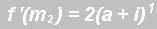OR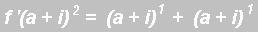Differentiation has separated this 2-dimensional complementation into its component parts in which the components are two 1-dimensional binaries. It has uncomplemented this complementation. This separation shown graphically is: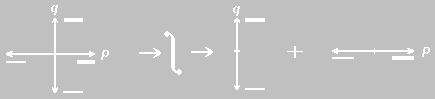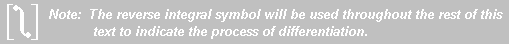The first derivative of this complementation is not the derivative of the active and inactive elements, it is the derivative of the complementation of two binary lines.

Integration of two 1-dimensional binary lines will produce a 2-dimensional complementation whose elements are cross multiplication products of the active and inactive 1-dimensional elements.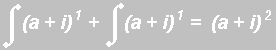OR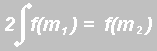The integration process is shown graphically below.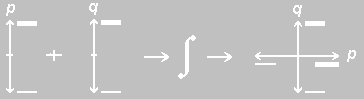The 0-dimension equation or Mension was previously defined as a vestige substance. The alchemists believed that this entity was the fuel for creation. The void, they believed, was an infinite sea of vestige entities (primordial soup) from which all things were created. Integration of a Mension (a singularity element from the primordial soup) will transform it from an inanimate vestige state to a 1-dimensional binary state.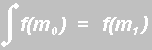OR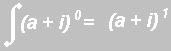This transformation is a transformation from the latent to the actual.